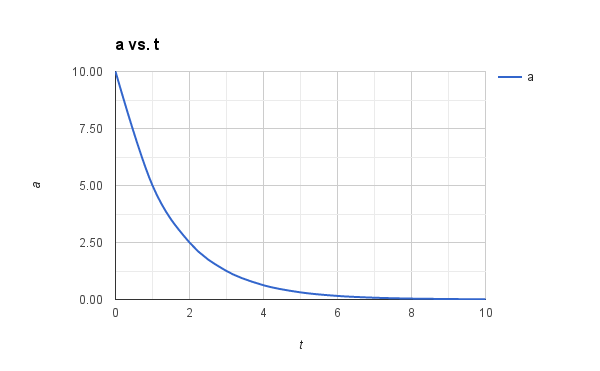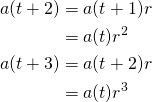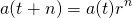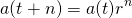# Frame Rate Independent Damping using Lerp

A quick aside for those not familiar with Unity: Each script has three different update functions that can be called. Update is called as you would expect, and LateUpdate is a version that’s called well, later on in the frame. Both of these should use the global (yikes) Time.deltaTime to access the variable frame time. FixedUpdate uses Time.fixedTimeDelta and runs on a fixed timestep, so can potentially run multiple times per frame.

## The Great Lerping Problem

It seems to crop up on the Unity forums again and again and again – the correct usage of linear interpolation for damping a value. You have a value, a, and you’d like to smoothly move it towards another value b, so you decide to use linear interpolation with some arbitrary rate r. If you do something like this in your variable rate update function (Update or LateUpdate), then you have a problem:

a = Mathf.Lerp(a, b, r);


This code is broken because it takes a chunk out between a and b each frame and we know that the frame rate is variable, so the smoothing will also be variable.

Once you figure this out, perhaps you do some research, and you find in the Unity docs that you should really be doing this:

a = Mathf.Lerp(a, b, r * Time.deltaTime);


Except, wait, this isn’t right either… The interpolation parameter can now potentially go over 1, which is not allowed. This is both wrong and even wronger. So what next?

Perhaps you decide that you should go back to the original lerp without deltaTime, but this time put it inside FixedUpdate instead.

I have some bad news for you… There is still a potential problem here. It’s a bit subtle, but things that run inside FixedUpdate are almost never at the correct state for the current frame since the update rates are different. This means that they require either extrapolation or interpolation to be displayed smoothly. Unity has an option to turn on extrapolation or interpolation for rigid bodies, so if you have this option on and you’re lerping a rigid body property then lerping will work as you would expect. However, if you’re lerping a value that isn’t extrapolated or interpolated then the smoothing is technically smooth as far as FixedUpdate is concerned, but you can still see stuttering on the screen.

One other problem that cannot be circumvented by using a fixed update is that if you need to change your update rate (for example you want to run physics at 100 Hz) and you use the plain lerp then your smoothing values will all need to be retuned.

## What Are We Trying To Do?

Let’s simplify things, and look at what we’re trying to actually achieve here. For now, let’s assume that we’re always interpolating towards zero.

One way of looking at this problem would be to ask how much of the initial value should be remaining after one second. Let’s say that we have an initial value of 10, and every second we would like to lose half of the current value:Let’s look at a graph of how this looks over time. We can see that it’s a nice and smooth curve going from our start value 10 down to almost zero. It will never quite reach zero, but it will get very close.Looking at the number sequence, we can generalize it pretty easily to:Or for an arbitrary rate rate r in the range (0, 1):What happens if we look more than one step ahead of the current value?I hope the pattern is clear here, so we can say even more generally:This means that we can take our value at our current time t and calculate the value for an arbitrary time in the future t + n. It’s crucial to realize here that n doesn’t have to be an integer value, so it’s quite fine to use deltaTime here. This means that we can now write a frame-rate aware function that will damp to zero and use it inside our variable rate update functions

// Smoothing rate dictates the proportion of source remaining after one second
//
public static float Damp(float source, float smoothing, float dt)
{
return source * Mathf.Pow(smoothing, dt);
}

private void Update()
{
a = Damp(a, 0.5f, Time.deltaTime);
}

// or

private void FixedUpdate()
{
a = Damp(a, 0.5f, Time.fixedDeltaTime);
}


I hear you – what if you want to go from a value a to a value b rather than to zero? The key thing to realise here is that it’s just a shift of the graph on the y-axis. If we’re now damping from 20 to 10 then it looks like this:So we need to add damp using (ab) and then add b back on afterwards. Let’s alter our damping function to do this:This should be looking pretty familiar… It’s in the same form as a standard Lerp but with an exponent on the rate parameter:

a(t + n) = Lerp(b, a(t), Pow(r, n))


You’ll probably notice here that the parameters are not in the order you might expect, but this is easy to fix since:

Lerp(a, b, t) = Lerp(b, a, 1 - t)


Therefore:

a(t + n) = Lerp(a(t), b, 1 - Pow(r, n))


We can write this code directly, or probably a better idea is to wrap it up into a function which will do frame-rate aware damping between two arbitrary values:

// Smoothing rate dictates the proportion of source remaining after one second
//
public static float Damp(float source, float target, float smoothing, float dt)
{
return Mathf.Lerp(source, target, 1 - Mathf.Pow(smoothing, dt))
}


A smoothing rate of zero will give you back the target value (i.e. no smoothing), and a rate of 1 is technically not allowed, but will just give you back the source value (i.e. infinite smoothing). Note that this is the opposite of the way a lerp parameter works, but if you so desire, you can just use additive inverse of the smoothing parameter inside the Pow.

## Exponential Decay

The keen-eyed among you may have looked at the graph and thought that it looks awfully like an exponential decay function. You would be right since it actually is an exponential decay function. To see why, let’s go back to the damping function without b in it:Now let’s compare this to the formula for exponential decay:Let’s equate these and see what happensThereforeSo an alternative way of expressing the damping function is to parameterize using lambda. This now has a range between zero and infinity, which nicely expresses the fact that you can never actually reach b when damping.

public static float Damp(float a, float b, float lambda, float dt)
{
return Mathf.Lerp(a, b, 1 - Mathf.Exp(-lambda * dt))
}


If you look around at other code, you’ll see the exponential decay form used commonly, but just know that it’s just another form of the frame-rate aware Lerp (or the other way around, depending on how you look at it).

Below is a graph showing both forms of damping with lambda calculated from the smoothing rate accordingly. As you can see, they both perfectly match.Finally, here is the same graph, but this time with a random time interval used.## Summary

• Don’t use plain Lerp for damping inside Update or FixedUpdate.
• Be careful about using Lerp inside FixedUpdate and be sure that the result will be interpolated or extrapolated.
• Prefer to use a frame-rate aware damping function when possible, even inside FixedUpdate since it will allow you to change the fixed update rate without retuning your damping.

I hope this clears up some of the confusion over how to use Lerp correctly when damping a value.

## 2 thoughts on “Frame Rate Independent Damping using Lerp”

1.Peter Pimley

A neat and little-known trick is that if you query Time.deltaTime during FixedUpdate, it will return the Time.fixedDeltaTime value . So generally you can use Time.deltaTime everywhere, and move code between FixedUpdate and Update without having to do a search-and-replace.

 http://docs.unity3d.com/ScriptReference/Time-deltaTime.html “When called from inside MonoBehaviour’s FixedUpdate, returns the fixed framerate delta time.”

(This is in version 5.3.1. It may have changed in the past and it may change in the future)

2.Matt Arrington

I’ve used the log method you describe. I would like to point out that multiplying by delta time should be ok! All the delta times should sum to 1.0 over a 1 second period, If not then something is seriously wrong with the game engine clock. A simple clamp is needed for the last frame that could potentially straddle the 1 second mark, (or what ever duration the leap occurs) Fixed update makes this more accurate as each time slice is the same value, and the leap should never lag even if there was a large processing spike and frame rate dropped .